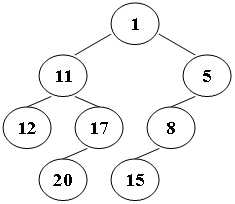PAT(A) 1127. ZigZagging on a Tree (30)

1127. ZigZagging on a Tree (30)

Suppose that all the keys in a binary tree are distinct positive integers. A unique binary tree can be determined by a given pair of postorder and inorder traversal sequences. And it is a simple standard routine to print the numbers in level-order. However, if you think the problem is too simple, then you are too naive. This time you are supposed to print the numbers in "zigzagging order" -- that is, starting from the root, print the numbers level-by-level, alternating between left to right and right to left. For example, for the following tree you must output: 1 11 5 8 17 12 20 15.Input Specification:

Each input file contains one test case. For each case, the first line gives a positive integer N (<= 30), the total number of nodes in the binary tree. The second line gives the inorder sequence and the third line gives the postorder sequence. All the numbers in a line are separated by a space.

Output Specification:

For each test case, print the zigzagging sequence of the tree in a line. All the numbers in a line must be separated by exactly one space, and there must be no extra space at the end of the line.

Sample Input: 8 12 11 20 17 1 15 8 5 12 20 17 11 15 8 5 1 Sample Output: 1 11 5 8 17 12 20 15

解题报告

1. 建树
2. 找出后序中最后一个元素在中序的位置，由这个位置将中序和后序一分为二，即两个序列左右部分元素个数相同。
3. 以后序最后一个元素为根，将分开的两部分依次按同样方法建立左右子树。
4. 输出，区分每一层的元素个数，判断向左还是向右，存入栈中，当一层进行完，再由栈进入队列中，保证顺序正确。

代码

/*
* Problem: 1127. ZigZagging on a Tree (30)
* Author: HQ
* Time: 2018-03-13
* State: Done
* Memo: 队列 栈 建树
*/
#include "iostream"
#include "queue"
#include "stack"
#include "vector"
using namespace std;

struct Node {
int data = 0;
struct Node * left = NULL;
struct Node * right = NULL;
};

int N;
vector<int> inorder, postorder;

int findPos(int x,int s,int l) {
for (int i = 0; i < l; i++) {
if (inorder[s + i] == x)
return s + i;
}
return -1;
}

struct Node * makeTree(int s1, int s2, int n) {
struct Node *root = NULL;
int x = postorder[s2 + n - 1];
int pos = findPos(x, s1, n);
if (pos != -1) {
root = new struct Node;
root->data = x;
if (pos - s1 > 0)
root->left = makeTree(s1, s2, pos - s1);
if (s1 + n - pos - 1> 0)
root->right = makeTree(pos + 1, s2 + pos - s1, s1 + n - pos - 1);
}
return root;
}

void levelOrder(struct Node * root) {
queue<struct Node *> q;
stack<struct Node *> s;
struct Node * temp;
q.push(root);
bool first = true;
bool left = false;
int cnt = 0, num = 1, tempnum = 0;
while (!q.empty()) {
temp = q.front();
q.pop();
if (first) {
cout << temp->data;
first = false;
}
else
cout << " " << temp->data;
if (left) {
if (temp->left != NULL) {
s.push(temp->left);
tempnum++;
}
if (temp->right != NULL) {
s.push(temp->right);
tempnum++;
}
}
else {
if (temp->right != NULL) {
s.push(temp->right);
tempnum++;
}
if (temp->left != NULL) {
s.push(temp->left);
tempnum++;
}
}
cnt++;
if (cnt == num) {
num = tempnum;
tempnum = 0;
cnt = 0;
left = !left;
while (!s.empty()) {
q.push(s.top());
s.pop();
}
}
}
}

int main() {
cin >> N;
inorder.resize(N);
postorder.resize(N);
for (int i = 0; i < N; i++)
cin >> inorder[i];
for (int i = 0; i < N; i++)
cin >> postorder[i];
struct Node * root;
root = makeTree(0, 0, N);
levelOrder(root);
cout << endl;
system("pause");
}